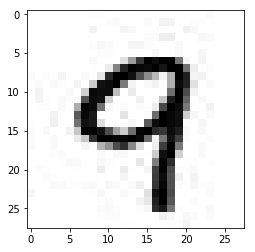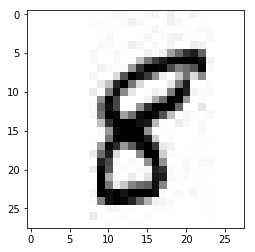Deep Learning Models -- A collection of various deep learning architectures, models, and tips for TensorFlow and PyTorch in Jupyter Notebooks.

In :
%load_ext watermark
%watermark -a 'Sebastian Raschka' -v -p tensorflow,numpy,h5py

Sebastian Raschka

CPython 3.6.1
IPython 6.0.0

tensorflow 1.2.0
numpy 1.13.0
h5py 2.7.0


# Storing an Image Dataset for Minibatch Training using HDF5¶

This notebook provides an example for how to save a large dataset of images as Hierarchical Data Format (HDF) for quick access during minibatch learning. This approach uses the common HDF5 format and should be accessible to any programming language or tool with an HDF5 API.

While this approach performs reasonably well (sufficiently well for my applications), you may also be interested in TensorFlow's "Reading Data" guide to work with TfRecords and file queues.

## 0. The Dataset¶

Let's pretend we have a directory of images containing two subdirectories with images for training, validation, and testing. The following function will create such a dataset of images in JPEG format locally for demonstration purposes.

In :
# Note that executing the following code
# and save all the 60,000 images as separate JPEG
# files. This might take a few minutes depending

import numpy as np

import sys
sys.path.insert(0, '..')
from helper import mnist_export_to_jpg

np.random.seed(123)
mnist_export_to_jpg(path='./')

Extracting ./train-images-idx3-ubyte.gz
Extracting ./train-labels-idx1-ubyte.gz
Extracting ./t10k-images-idx3-ubyte.gz
Extracting ./t10k-labels-idx1-ubyte.gz

In :
import os

for i in ('train', 'valid', 'test'):
print('mnist_%s subdirectories' % i, os.listdir('mnist_%s' % i))

mnist_train subdirectories ['.DS_Store', '0', '1', '2', '3', '4', '5', '6', '7', '8', '9']
mnist_valid subdirectories ['0', '1', '2', '3', '4', '5', '6', '7', '8', '9']
mnist_test subdirectories ['0', '1', '2', '3', '4', '5', '6', '7', '8', '9']


Note that the names of the subdirectories correspond directly to the class label of the images that are stored under it.

To make sure that the images look okay, the snippet below plots an example image from the subdirectory mnist_train/9/:

In :
%matplotlib inline
import matplotlib.image as mpimg
import matplotlib.pyplot as plt
import os

some_img = os.path.join('./mnist_train/9/', os.listdir('./mnist_train/9/'))

print(img.shape)
plt.imshow(img, cmap='binary');

(28, 28)Note: The JPEG format introduces a few artifacts that we can see in the image above. In this case, we use JPEG instead of PNG. Here, JPEG is used for demonstration purposes since that's still format many image datasets are stored in.

## 1. Saving images as HDF5¶

The following wrapper function creates .h5 file containing training, testing, and validation datasets. It will group images together into larger integer arrays that are then saved as subgroups in the HDF5 file. For instance, the training images will be saved as 'train/images' and the corresponding labels as 'train/labels' subgroup.

In :
import numpy as np
import h5py
import glob

def images_to_h5(data_stempath='./mnist_',
width=28, height=28, channels=1,
shuffle=False, random_seed=None):

with h5py.File('mnist_batches.h5', 'w') as h5f:

for s in ['train', 'valid', 'test']:
img_paths = [p for p in glob.iglob('%s%s/**/*.jpg' %
(data_stempath, s),
recursive=True)]

dset1 = h5f.create_dataset('%s/images' % s,
shape=[len(img_paths),
width, height, channels],
compression=None,
dtype='uint8')
dset2 = h5f.create_dataset('%s/labels' % s,
shape=[len(img_paths)],
compression=None,
dtype='uint8')
dset3 = h5f.create_dataset('%s/file_ids' % s,
shape=[len(img_paths)],
compression=None,
dtype='S5')

rand_indices = np.arange(len(img_paths))

if shuffle:
rng = np.random.RandomState(random_seed)
rng.shuffle(rand_indices)

for idx, path in enumerate(img_paths):

rand_idx = rand_indices[idx]
label = int(os.path.basename(os.path.dirname(path)))
dset1[rand_idx] = image.reshape(width, height, channels)
dset2[rand_idx] = label
dset3[rand_idx] = np.array([os.path.basename(path)], dtype='S6')


Note that we didn't specify any compression format. The reason is that non-compressed HDF5 datasets are much faster to read, which is an important factor for training deep learning systems. In this case, the dataset is about ~47 Mb in size. However, we are working with larger datasets, compressing the HDF5 dataset might be one easy way to deal with hardware storage limitations.

In :
images_to_h5(shuffle=True, random_seed=123)


To check that the archiving worked correctly, we will now load the training images and print the array shape. Note that we can now access each archive similar to a python dictionary. Here the 'data' key contains the image data and the 'labels' key stores an array containing the corresponding class labels:

In :
with h5py.File('mnist_batches.h5', 'r') as h5f:
print(h5f['train/images'].shape)
print(h5f['train/labels'].shape)
print(h5f['train/file_ids'].shape)

(45000, 28, 28, 1)
(45000,)
(45000,)

In :
with h5py.File('mnist_batches.h5', 'r') as h5f:

plt.imshow(h5f['train/images'][:, :, -1], cmap='binary');
print('Class label:', h5f['train/labels'])
print('File ID:', h5f['train/file_ids'])

Class label: 8
File ID: b'20829'The following cell implements a class for iterating over the MNIST images, based on the .h5 file, conveniently. Via the normalize parameter we additionally scale the image pixels to [0, 1] range, which typically helps with gradient-based optimization in practice.

The key functions (here: generators) are

These let us iterate over small chunks (determined via minibatch_size) and yield minibatches via memory-efficient Python generators. Via the two shuffle parameters, we can further control if the images within each batch to be shuffled. By setting onehot=True, the labels are converted into a onehot representation for convenience.

In :
class BatchLoader():
def __init__(self, minibatches_path,
normalize=True):

self.minibatches_path = minibatches_path
self.normalize = normalize
self.num_train = 45000
self.num_valid = 5000
self.num_test = 10000
self.n_classes = 10

shuffle_batch=False, prefetch_batches=1, seed=None):
batch_size=batch_size,
onehot=onehot,
shuffle_batch=shuffle_batch,
prefetch_batches=prefetch_batches,
seed=seed):
yield batch_x, batch_y

shuffle_batch=False, prefetch_batches=1, seed=None):
batch_size=batch_size,
onehot=onehot,
shuffle_batch=shuffle_batch,
prefetch_batches=prefetch_batches,
seed=seed):
yield batch_x, batch_y

shuffle_batch=False, prefetch_batches=1, seed=None):
batch_size=batch_size,
onehot=onehot,
shuffle_batch=shuffle_batch,
prefetch_batches=prefetch_batches,
seed=seed):
yield batch_x, batch_y

shuffle_batch=False, prefetch_batches=1, seed=None):

prefetch_size = prefetch_batches * batch_size

if shuffle_batch:
rgen = np.random.RandomState(seed)

with h5py.File(self.minibatches_path, 'r') as h5f:
indices = np.arange(h5f['%s/images' % which].shape)

for start_idx in range(0, indices.shape - prefetch_size + 1,
prefetch_size):

x_batch = h5f['%s/images' % which][start_idx:start_idx + prefetch_size]
x_batch = x_batch.astype(np.float32)
y_batch = h5f['%s/labels' % which][start_idx:start_idx + prefetch_size]

if onehot:
y_batch = (np.arange(self.n_classes) ==
y_batch[:, None]).astype(np.uint8)

if self.normalize:
# normalize to [0, 1] range
x_batch = x_batch.astype(np.float32) / 255.

if shuffle_batch:
rand_indices = np.arange(prefetch_size)
rgen.shuffle(rand_indices)
x_batch = x_batch[rand_indices]
y_batch = y_batch[rand_indices]

for batch_idx in range(0, x_batch.shape - batch_size + 1,
batch_size):

yield (x_batch[batch_idx:batch_idx + batch_size],
y_batch[batch_idx:batch_idx + batch_size])


The following for loop will iterate over the 45,000 training examples in our MNIST training set, yielding 50 images and labels at a time (note that we previously set aside 5000 training example as our validation datast).

In :
batch_loader = BatchLoader(minibatches_path='./mnist_batches.h5',
normalize=True)

print(batch_x.shape)
print(batch_y.shape)
break

(50, 28, 28, 1)
(50, 10)

In :
cnt = 0
batch_size=100, onehot=True):
cnt += batch_x.shape

print('One training epoch contains %d images' % cnt)

One training epoch contains 45000 images

In :
def one_epoch():
batch_size=100, onehot=True):
pass

% timeit one_epoch()

323 ms ± 9.98 ms per loop (mean ± std. dev. of 7 runs, 1 loop each)


As we can see from the benchmark above, an iteration over one training epoch (45k images) is relatively fast.

Similarly, we could iterate over validation and test data via

Note that increasing the batch_size can substantially improve the computationally efficiency loading an epoch, since it would lower the number of iterations. Further, we used two nested for loops in _load_epoch, where the inner one yields the actual batches. The purpose of the outer loop in this function is to prefetch multiple batches for shuffling -- otherwise, the shuffling won't have any effect on the gradients.

In :
def one_epoch():
batch_size=100, shuffle_batch=True, prefetch_batches=4,
seed=123, onehot=True):
pass

% timeit one_epoch()

172 ms ± 11.8 ms per loop (mean ± std. dev. of 7 runs, 10 loops each)


Also, as we can see from the benchmark, prefetching multiple batches from the HDF5 database can speed up the loading of an epoch. Note that this could not always practicable (for example, when we are working with high-resolution images) due to memory constraints.

## 3. Training a Model using TensorFlow's feed_dict¶

The following code demonstrate how we can feed our minibatches into a TensorFlow graph using a TensorFlow session's feed_dict.

### Multilayer Perceptron Graph¶

In :
import tensorflow as tf

##########################
### SETTINGS
##########################

# Hyperparameters
learning_rate = 0.1
training_epochs = 15
batch_size = 100

# Architecture
n_hidden_1 = 128
n_hidden_2 = 256
height, width = 28, 28
n_classes = 10

##########################
### GRAPH DEFINITION
##########################

g = tf.Graph()
with g.as_default():

tf.set_random_seed(123)

# Input data
tf_x = tf.placeholder(tf.float32, [None, height, width, 1], name='features')
tf_x_flat = tf.reshape(tf_x, shape=[-1, height*width])
tf_y = tf.placeholder(tf.int32, [None, n_classes], name='targets')

# Model parameters
weights = {
'h1': tf.Variable(tf.truncated_normal([width*height, n_hidden_1], stddev=0.1)),
'h2': tf.Variable(tf.truncated_normal([n_hidden_1, n_hidden_2], stddev=0.1)),
'out': tf.Variable(tf.truncated_normal([n_hidden_2, n_classes], stddev=0.1))
}
biases = {
'b1': tf.Variable(tf.zeros([n_hidden_1])),
'b2': tf.Variable(tf.zeros([n_hidden_2])),
'out': tf.Variable(tf.zeros([n_classes]))
}

# Multilayer perceptron
layer_1 = tf.nn.relu(layer_1)
layer_2 = tf.nn.relu(layer_2)
out_layer = tf.matmul(layer_2, weights['out']) + biases['out']

# Loss and optimizer
loss = tf.nn.softmax_cross_entropy_with_logits(logits=out_layer, labels=tf_y)
cost = tf.reduce_mean(loss, name='cost')
train = optimizer.minimize(cost, name='train')

# Prediction
correct_prediction = tf.equal(tf.argmax(tf_y, 1), tf.argmax(out_layer, 1))
accuracy = tf.reduce_mean(tf.cast(correct_prediction, tf.float32), name='accuracy')


### Training the Neural Network with Minibatches¶

In :
##########################
### TRAINING & EVALUATION
##########################

normalize=True)

# by unpacking the generator
onehot=True)
valid_x, valid_y = valid_data, valid_data
del valid_data

with tf.Session(graph=g) as sess:
sess.run(tf.global_variables_initializer())

for epoch in range(training_epochs):
avg_cost = 0.

n_batches = 0
onehot=True,
shuffle_batch=True,
prefetch_batches=10,
seed=epoch):
n_batches += 1
_, c = sess.run(['train', 'cost:0'], feed_dict={'features:0': batch_x,
'targets:0': batch_y.astype(np.int)})
avg_cost += c

train_acc = sess.run('accuracy:0', feed_dict={'features:0': batch_x,
'targets:0': batch_y})

valid_acc = sess.run('accuracy:0', feed_dict={'features:0': valid_x,
'targets:0': valid_y})

print("Epoch: %03d | AvgCost: %.3f" % (epoch + 1, avg_cost / n_batches), end="")
print(" | MbTrain/Valid ACC: %.3f/%.3f" % (train_acc, valid_acc))

# imagine test set is too large to fit into memory:
test_acc, cnt = 0., 0
onehot=True):
cnt += 1
acc = sess.run(accuracy, feed_dict={'features:0': test_x,
'targets:0': test_y})
test_acc += acc
print('Test ACC: %.3f' % (test_acc / cnt))

Epoch: 001 | AvgCost: 0.462 | MbTrain/Valid ACC: 0.950/0.901
Epoch: 002 | AvgCost: 0.218 | MbTrain/Valid ACC: 0.980/0.935
Epoch: 003 | AvgCost: 0.164 | MbTrain/Valid ACC: 0.980/0.950
Epoch: 004 | AvgCost: 0.132 | MbTrain/Valid ACC: 1.000/0.958
Epoch: 005 | AvgCost: 0.111 | MbTrain/Valid ACC: 1.000/0.958
Epoch: 006 | AvgCost: 0.096 | MbTrain/Valid ACC: 0.980/0.964
Epoch: 007 | AvgCost: 0.083 | MbTrain/Valid ACC: 0.990/0.965
Epoch: 008 | AvgCost: 0.073 | MbTrain/Valid ACC: 0.990/0.966
Epoch: 009 | AvgCost: 0.064 | MbTrain/Valid ACC: 1.000/0.966
Epoch: 010 | AvgCost: 0.057 | MbTrain/Valid ACC: 0.980/0.967
Epoch: 011 | AvgCost: 0.050 | MbTrain/Valid ACC: 1.000/0.969
Epoch: 012 | AvgCost: 0.045 | MbTrain/Valid ACC: 1.000/0.969
Epoch: 013 | AvgCost: 0.040 | MbTrain/Valid ACC: 0.990/0.971
Epoch: 014 | AvgCost: 0.036 | MbTrain/Valid ACC: 1.000/0.969
Epoch: 015 | AvgCost: 0.032 | MbTrain/Valid ACC: 1.000/0.970
Test ACC: 0.974